# Information Theory and Creationism

## Classical Information Theory (Shannon)

by
[Posted: July 14, 2005]### Claude Shannon and Classical Information Theory  [Top]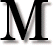odern digital communication depends on Information Theory, which was invented in the 1940's by Claude E. Shannon. Shannon first published A Mathematical Theory of Communication in 1947-1948, and jointly published The Mathematical Theory of Communciation with Warren Weaver in 1949. That text is still in publication by the University of Illinois Press. Information Theory, sometimes referred to as Classical Information Theory as opposed to Algorithmic Information Theory, provides a mathematical model for communication. Though Shannon was principally concerned with the problem of electronic communications, the theory has much broader applicability. Communication occurs whenever things are copied or moved from one place and/or time to another.

This article briefly describes the main concepts of Shannon's theory. The mathematical proofs are readily available in many sources, including the Internet links on this page. While Shannon's theory covers both digital and analog communication, analog communication will be ignored for simplicity. On the other hand, Information Theory is a fairly technical subject, generally introduced to third-year engineering university students. Really understanding it requires knowledge of statistics and calculus.

For those who wonder how a theory about communication can possibly relate to biological evolution, a visit to Tom Schneider's web site, Molecular Information Theory and the Theory of Molecular Machines, may help. In any case, Creationists are now fond of arguing about information, and this article provides useful background material on the subject.

### Information Sources [Top]

An information source is a system that outputs from a fixed set of M symbols {a1 .. aM} in a sequence at some rate (see Fig. 1). In the simplest case, each symbol that might be output from the system is equally likely. The letter i will stand for some given ouput symbol from the set {a1 .. aM}. If all symbols are equally likely, then the probability that symbol i will be the one produced is p1/M no matter which symbol we have in mind. For example, if the information source can produce four equally likely symbols (A, B, C, and D), then each symbol has a probability of .25 (that is, 25% or 1/4).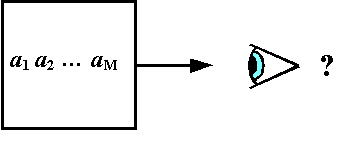An observer is uncertain which of the M symbols will be output. Once a given symbol ai is observed, the observer has obtained information from the source. The observer's uncertainty is reduced. The amount of information obtained can be measured because the number of possible symbols is known. By definition, the measure of the information I observed from the system is given by:

I =  log2 = -logP,

and the unit of measure is binary digits, or bits. The unit of measure depends on the base of the logarithm. Most of the time, Information Theory uses the base 2 logarithm (log2). Any other logarithm base would work. If we used base 10, then the unit of measure would be decimal digits.

If a system can output any of 16 possible symbols, for each symbol observed the observer receives 4 bits of information. That is, it reduces the observer's uncertainty by 4 bits (see Fig. 2). The use of a logarithm to measure information is owed to Ralph V.L. Hartley whose 1928 paper is cited by Shannon.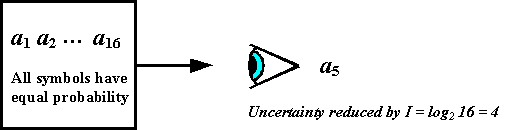It is easy to see the utility in this definition. When an information source capable of outputing any one of the 128 basic ASCII symbols, all equally likely, produces a symbol, the observer obtains 7 bits of information, exactly the number of bits used to encode the basic ASCII symbol set. (ASCII stands for American Standard Code for Information Interchange, in use for many years in teletype machines and computers. Of course an actual ASCII information source does not produce 128 equally likely symbols, but for simplicity we will pretend it does right now.)Shannon describes a communication system as an information source, transmitter, channel, receiver, and destination (see Fig. 3). The destination is our observer. A basic requirement for a communication system is that, with high reliability, the symbol at the information source and the symbol at the destination match. Shannon had a key insight regarding this: it doesn't matter whether the symbol means anything. It only matters whether the symbol at the information source and the destination are the same. It was this insight, meaning must be ignored, that enabled Shannon to create a workable mathematical model for information.

Next, consider that symbols output by an information source might not be equally probable. Suppose the symbols {a1 .. aM} occur with probabilities p.. pM. Since {a1 .. aM} are all the possible symbols, the sum of probabilities pi over all i is 1. Because the observer doesn't know what the next symbol in the output sequence will be, the information source is treated as a stochastic process. That is, we assume the symbols output are random, occuring with the given probabilities.

A simple example of a stochastic process is a person tossing a coin over and over again (see Fig. 4). The results are sometimes heads and sometimes tails, but we can't predict the results for any given toss of the coin. We assume, of course, that the coin is a fair coin, which has a 50% chance of heads and a 50% chance of tails when tossed. If we recorded the sequence of coin tosses, it might look like HHTHTHHTHTTTTHTHHT...The next concept needed is a finite state machine. This is a kind of machine that has a finite number of conditions or states that it can be in at any given point in time. A light switch, for example, has two states- ON and OFF. A finite state machine does not need to physically exist. It might simply be a mathematical model on paper or in a computer. The machine's state can be recorded as time goes on, producing a sequence just like the coin toss. If we recorded the position of a light switch in the bedroom of a hyperactive child every 15 minutes, it might even look rather random, like ON - ON - OFF - ON - OFF - ON - ON - OFF - ON - OFF - OFF - OFF - OFF - ON - OFF - ON - ON - OFF... just like tossing a coin.

We can now model an information source as a kind of random state machine, hopping about from state to state, with each state corresponding to the next output symbol. We can still give the information received by observing symbol i in terms of its probability:

I= -logpi

Thinking about the information source this way, it is reasonable to suppose that the probability of its next state will depend on its current state. For example, a light switch in the bedroom of a sedentary octogenarian would probably not change much at 15 minute intervals: ON - ON - ON - ON - ON - ON - OFF - OFF - OFF - OFF...

Let's consider a single state change. Our state machine might change from state i to state j. That state change has a certain probability. We can describe the probability that j follows i as p(i). The notation p(i) is read aloud as "the probability of j given i." If you read Shannon's article, note that he uses a less conventional notation pi(j) rather than p(i).

To keep things simple, we are not going to let our state machine remember what its state used to be. It only knows what state it is in now. That means the probability of its next state can only depend on the current state. It can't depend on how the machine got in the current state. If we then look at all the possible state transistions for the machine, we can write down the set of state transition probabilities. For each symbol i the sum of all p(i) over j is 1. The set of state transition probabilities for our light switch is:

{ p(ON ON), p(ONOFF), p(OFF ON), p(OFF OFF) }

It is easy to see that p(ON ON) + p(OFFON) = 1, and also that p(ON OFF) + p(OFFOFF) = 1.

A stochastic process of this type, where the probability distribution of the next state depends on the current state but nothing earlier, is called a discrete Markov process. Shannon represents information sources as discrete Markov processess. That is, the information source is assumed to be a random process, outputing a sequence of symbols from a fixed symbol set, where the probability of each new symbol depends on only on the preceding symbol. The state machine cannot remember anything before that.

An ergodic process is a special class of Markov process. For an ergodic process, every sequence it can produce is the same in statistical properties. If the information source is ergodic, then the probability that symbol j occurs after N symbols, Pj(N), converges to an equilibrium value with increasingly large N. Shannon makes a general assumption that information sources are ergodic processes.

### Information, Entropy, and Uncertainty [Top]

Remember that the observer begins completely uncertain what sequence of symbols will be output from the information source. As each symbol is observed, the uncertainty decreases. We can represent the information source as a random variable, since its state might match any value in the symbol set, and we'll call it X just like Shannon does. The random variable X can produce any of the M symbols {a1 .. aM}. Each of these symbols has a probability p1 .. pM , and those probabilities must all add up to 1.

The information received from X when it produces a symbol i is

I= -log2pi

The entropy of X is defined by its average information:

H(X) = E{ I} = -∑  i plogp)

The notation E{ I} denotes the expected value of IX, which is a more specific term in statistics than “average”. Entropy can also be called average uncertainty (strictly speaking, the average reduction in uncertainty for a receiver).

Why is information entropy defined as this weighted sum of probabilities? Mainly because the probabilities associated with symbols are not, in general, equal. Consider the 26 letters of the English alphabet. As Shannon noted, the letter “E” is occurs more frequently than “Q”, the sequence “TH” more than “XP”, etc. By defining entropy as the average information, it will tell us something about how fully a communication channel is being used.

The entropy function H(X) is maximum when p1/M for all i. This makes intuitive sense, because uncertainty is greatest when all outcomes are equally likely. On the other hand, if an information source produces symbol a1 with probability .999 and ten other symbols a.. a11 each with probability .0001, we are nearly certain that the next symbol will be a1.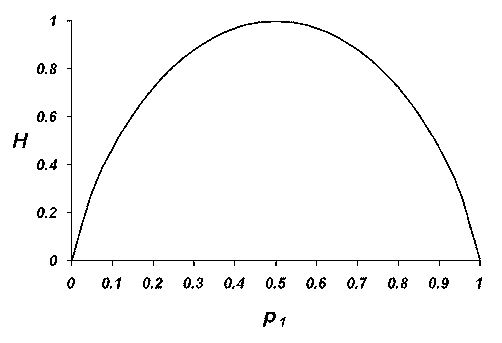Consider a binary information source capable of producing symbols 0 and 1. Its entropy as a function of the probability p1 of symbol 1 is shown on Fig. 5. (Remember that p0  = 1-p1 because there are only two symbols allowed and there probabilities must sum to 1). Note that the entropy reaches a maximum of 1 when pp= 1/2. When both symbols are equally likely, the observer is most uncertain. The sequence of heads or tails from multiple tosses of a fair coin, for example, has maximum entropy. On the other hand, if the information source always produced symbol 1 with probability p= 1, the entropy and the observer's uncertainty are zero. If the information source never produced symbol 1 but always produced symbol 0, the entropy and the observer's uncertainty are likewise zero. No information is gained by observing an event known to never change.

### Conditional and Joint Entropy [Top]

If X and Y are random variables representing the input and output of a channel, respectively, then the conditional entropy (meaning the average uncertainty of the received symbol given that X was transmitted) is:

H(X) = -∑ i, j  p(xiyj) log p(yj xi),

the joint entropy (meaning the average uncertainty of the total information system) is:

H(XY) = -∑ i, j  p(xiyj) log2   p(xiyj),

and the equivocation entropy (meaning the average uncertainty of the transmitted symbol after a symbol is received) is:

H(Y) = -∑ i, j  p(xiyj) log2   p(xyj).

The notation p(A, B) means the probability of A and B both occurring, while p(A | B) means the probability of A occurring given that B has occurred.

An important relationship is:

H(XY) = H(Y) +  H(Y) = H(X) + H( X).

### Implications for Communication [Top]

Shannon demonstrated that, for a channel with capacity C and an information source with entropy H, it is possible to transmit the encoded output of the information source through the channel at an average rate of up to (C/H)  - e, where e is an arbitrarily small number. It is not possible to transmit at an average rate exceeding C/H.

Shannon and R.M. Fano independently developed an efficient encoding method, known as the Shannon-Fano technique, in which codeword length increases with decreasing probability of source symbol or source word. The basic idea is that frequently used symbols (like the letter E) should be coded shorter than infrequently used symbols (like the letter Z) to make best use of the channel, unlike ASCII coding where both E and Z require the same seven bits.

If communication occurs with input X and output Y, the capacity of a channel is:

C = Max ( H(X) - H(X | Y) )

The function Max(H) means the maximum value of H. For a noiseless channel, H(Y) = 0. Since H(X) is maximal when all M possible symbols for X are equally probable, = logM, or I(X).

Shannon also provided the mathematical basis for transmitting on a channel with noise (see Fig. 6). A noisy channel can be seen as connected to two stochastic processes- the information source and the noise source.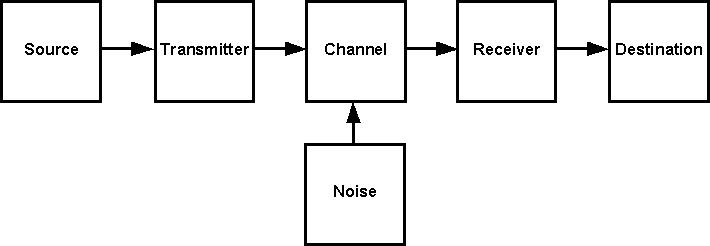If X is the information source and Y is the received information, then by using a new channel (a correction channel) with capacity equal to the conditional entropy H(X), it is possible to encode the correction data so that all but an arbitrarily small fraction e of the errors can be corrected (see Fig. 7). If the entropy of the information source H ≤ C, then the information source can be transmitted with an arbitrarily small error rate. If C then no method of encoding is possible such that the equivocation is less than H-C.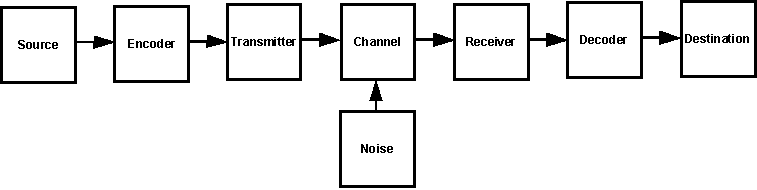There are many examples in the literature of error correction codes. Some of the more well known are the Hamming, Reed-Solomon, Viterbi, and Fire codes.

### Information and Noise [Top]

An important point to bear in mind is that both the information source and the noise source on Fig. 6 and Fig. 7 are stochastic processes. They could both be treated as information sources. The main difference between them is that the receiver is interested in the information source and wants to ignore the noise source.

In some situations a noise source is intentionally observed, in which case it becomes an information source. On the other hand, sometimes information is copied unintentionally from one channel into another one. This is called crosstalk, and its result is that someone else's uninteresting information source is considered noise.

When scientists measure cosmic background radiation to study it, it is information. Interference in a communication system from cosmic background radiation is noise. Terrain elevation data can be considered random. If you record it to make a topographic map, it is information. If you want to measure the diameter of a planet, it is noise. This concept, that one person's information is another person's noise and vice-versa, is commonly misunderstood in lay discussions about Information Theory. To reiterate, it is the interest of the observer that turns a stochastic process into an information source.

Some creationists will argue that noise degrades information just like the Second Law of Thermodynamics degrades order, and imply that the Second Law applies to information. But for Shannon’s definition of information, since we don’t care about meaning, and since the difference between information and noise depends only on our interest, this argument holds no water. We change noise into information simply by deciding to care about it, and information into noise by choosing to ignore it. Further, thermodynamic order is about the arrangement of molecules and similar objects, not mathematical symbols, so their argument is doubly wrong. Information symbols are mathematical abstractions, and need not behave like any particular physical system (such as a collection of molecules), as will be seen in the next section.

Some creationists also argue for a Law of Conservation of Information, similar to the First Law of Thermodynamics. We must remember that Conservation of Energy is a principle established by more than 150 years of scrupulous data collection, and there is no rigor whatsoever behind the notion of Conservation of Information. In any case, under Shannon’s definition of information this proposed law is nonsense. All you need to create information is an ergodic process and an observer to watch it. Atomic decay provides a simple physical example. An observer of atomic decay gets new information (which atom, what time, what products) as each atom decays.

### Shannon Entropy vs. Thermodynamic Entropy [Top]

Shannon's information entropy function has exactly the same form as the equation of Boltzmann's H-Theorem:

H(t) = ∫ f ln f dc

where ∫  is the integral symbol from calculus, ln means natural (base e) logarithm, f is the distribution function for molecules in an ideal gas, and c is the velocity space. The symbol H is used in Information Theory because of this similarity.

Interestingly, Brownian motion (the random thermal motion of molecules) is also a Markov process. It is from the H(t) formula that we can derive:

S = k ln w

where S is the thermodynamic entropy of a system, k is Boltzmann's constant, and w is the disorder of the system; that is, the probability that a system will exist in the state it is in relative to all the possible states it could be in. Boltzmann's H-Theorem tells us that, after a long time, f will reach equilibrium. This is similar to what Shannon tells us about information sources modeled as ergodic processes. Despite the similarities, Shannon entropy and thermodynamic entropy are not the same. Thermodynamic entropy characterizes a statistical ensemble of molecular states, while Shannon entropy characterizes a statistical ensemble of messages.

In thermodynamics, entropy has to do with all the ways the molecules or particles might be arranged, and greater entropy means less physical work can be extracted from the system. In Shannon’s usage, entropy has to do with all the ways messages might be transmitted by an information source, and greater entropy means the messages are more equally probable. Entropy in information theory does not mean information is becoming more useless or degraded; and because it is a mathematical abstraction, it does not directly relate to physical work unless you are treating molecules informatically.

Shannon entropy has been related by physicist Léon Brillouin to a concept sometimes called negentropy. This is a term introduced by physicist and Nobel laureate Erwin Schrödinger in his 1944 text What is Life to explain how living systems export entropy to their environment while maintaining themselves at low entropy; in other words, it is the negative of entropy. In his 1962 book Science and Information Theory, Brillouin described the Negentropy Principle of Information or NPI, the gist of which is that acquiring information about a system’s microstates is associated with a decrease in entropy (work is needed to extract information, erasure leads to increase in thermodynamic entropy). There is no violation of the Second Law of Thermodynamics involved, since a reduction in any local system’s thermodynamic entropy results in an increase in thermodynamic entropy elsewhere.

The relationship between the Shannon's information entropy H and the entropy S from statistical mechanics was given more rigor by Edwin Jaynes in 1957. The upshot is that information entropy and thermodynamic entropy are closely related metrics, but are not the same metric. For most practioners of Information Theory up to now, this poses no difficulty, because their field is communication and computation using conventional electronic circuits where the thermodynamic meaning of entropy is not discussed. The conflicting terminology results in much confusion, however, in areas like molecular machines and physics of computation, where information and thermodynamic entropy are dealt with side by side. Some authors, like Tom Schneider, argue for dropping the word entropy for the H function of Information Theory and using Shannon's other term uncertainty (average surprisal) instead. For more on this see Information Is Not Entropy, Information Is Not Uncertainty!

Unlike molecular entropy, Shannon entropy can be locally reduced without putting energy into the information system. Simply passing a channel through a passive filter can reduce the entropy of the transmitted information (unbeknownst to the transmitter, the channel capacity is reduced, and therefore so is the entropy of the information on the channel). The amount of power needed to transmit is the same whether or not the filter is in place, and whether or not the information entropy is reduced. Another way to think about this is to cut one wire of a channel having multiple parallel wires. The average information going across the channel, the entropy, goes down, with no relationship to the amount of energy needed to cut the wire. Or shut off the power supply to an information source and watch its output fix on one single symbol “off” with probability 1 and information entropy 0.

A word of caution about notation: for those familiar with chemical thermodynamics, H in Classical Information Theory is not enthalpy, and the two subjects should not be confused. Similarly, the H in Classical Information Theory measures a different property than the H in Algorithmic Information Theory. While these conflicts in notation may be regrettable, all the literature is written that way and anyone wishing to understand Information Theory will simply have to get used to it.

[Top]Home Page | Browse | Search | Feedback | Links The FAQ | Must-Read Files | Index | Creationism | Evolution | Age of the Earth | Flood Geology | Catastrophism | Debates